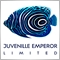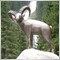# If an EA uses an indicator that is drawn on the chart, should it also be drawn on the Strategy Tester?246

I have an EA that I use which uses an indicator that is drawn on the chart, but when I run it in the strategy tester, it doesn't appear on the screen except for some small objects. Is it supposed to be drawn on the Strategy Tester chart or is it not supposed to be showing?

If it is supposed to be showing on the Strategy Tester screen, how can I make it so?

Thank you!Moderator
18963

JingleJongle:

I have an EA that I use which uses an indicator that is drawn on the chart, but when I run it in the strategy tester, it doesn't appear on the screen except for some small objects. Is it supposed to be drawn on the Strategy Tester chart or is it not supposed to be showing?

If it is supposed to be showing on the Strategy Tester screen, how can I make it so?

Thank you!

If the indicator's contribution is calculated in your tester's results, its not absolute that it should be drawn on the chart too.57

JingleJongle:

I have an EA that I use which uses an indicator that is drawn on the chart, but when I run it in the strategy tester, it doesn't appear on the screen except for some small objects. Is it supposed to be drawn on the Strategy Tester chart or is it not supposed to be showing?

If it is supposed to be showing on the Strategy Tester screen, how can I make it so?

Thank you!

Hello JingleJongle,

Problem: I initailized the indicator within the global section of my EA. This works but the Indicator dosn't show up in Strategy Tester. Example:

```input    int                  SMA_PERIOD             = 200;
input    int                  SMA_SHIFT              = 0;
input    ENUM_TIMEFRAMES      SMA_TIMEFRAME          = PERIOD_CURRENT;
input    ENUM_MA_METHOD       SMA_METHOD             = MODE_SMA;
input    ENUM_APPLIED_PRICE   SMA_PRICE              = PRICE_CLOSE;

int   hSMA = iMA(_Symbol, SMA_TIMEFRAME, SMA_PERIOD, SMA_SHIFT, SMA_METHOD, SMA_PRICE);

int OnInit()
{
return(INIT_SUCCEEDED);
}```

Solution: Make sure your Indicator is initialized within the OnInit() section of your EA. In my case the MA line now showed up within the Strategy Tester.

```input    int                  SMA_PERIOD             = 200;
input    int                  SMA_SHIFT              = 0;
input    ENUM_TIMEFRAMES      SMA_TIMEFRAME          = PERIOD_CURRENT;
input    ENUM_MA_METHOD       SMA_METHOD             = MODE_SMA;
input    ENUM_APPLIED_PRICE   SMA_PRICE              = PRICE_CLOSE;

int   hSMA = INVALID_HANDLE;

int OnInit()
{
hSMA =  iMA(_Symbol, SMA_TIMEFRAME, SMA_PERIOD, SMA_SHIFT, SMA_METHOD, SMA_PRICE);

return(INIT_SUCCEEDED);
}
```